### Home > PC > Chapter 4 > Lesson 4.1.4 > Problem4-54

4-54.
1.Roger is in an Algebra 2 class and has found the radian setting on his calculator. He has not learned about radians but would like to know. Homework Help ✎

1. Explain to Roger how a radian is defined.

2. Show him how to change 240° to radians.

3. Show him how to change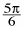radians to degrees.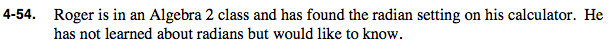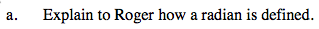1 radian is the angle measure found by wrapping one radius around a circle.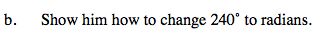$\frac{\pi\text{ radians}}{180\degree}$

Use a Giant One of

$240\degree\cdot\frac{\pi\text{ radians}}{180\degree}$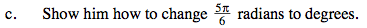$\frac{5\pi}{6}\cdot \frac{180\degree}{\pi}=?$